# NEET Previous Year Questions (2014-21): Electromagnetic Waves Notes | Study Physics Class 12 - NEET

## NEET: NEET Previous Year Questions (2014-21): Electromagnetic Waves Notes | Study Physics Class 12 - NEET

The document NEET Previous Year Questions (2014-21): Electromagnetic Waves Notes | Study Physics Class 12 - NEET is a part of the NEET Course Physics Class 12.
All you need of NEET at this link: NEET

Q.1. For a plane electromagnetic wave propagating in the x-direction, which one of the following combinations gives the correct possible directions for the electric field (E) and magnetic field (B) respectively?     (2021)
A: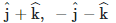B: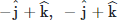C: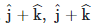D: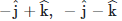Ans: DQ.2. A capacitor of capacitance 'C' is connected across an ac source of voltage V, given by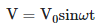The displacement current between the plates of the capacitor would then be given by:   (2021)
A: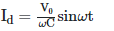B: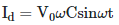C: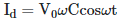D: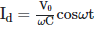Ans: CQ.3. The ratio of contributions made by the electric field and magnetic field components to the intensity of an electromagnetic wave is : (c = speed of electromagnetic waves)     (2020)
A: 1 : c
B: 1 : c2
C: c : 1
D: 1 : 1

Ans: D
Energy distribution is same so ration of electric field and magnetic field will be 1 : 1

Q.4. Which colour of the light has the longest wavelength?    (2019)
A: Red
B: Blue
C: Green
D: Violet
Ans: A
Solution:

Red has the longest wavelength among the given options.

Q.5. An em wave is propagating in a medium with a velocity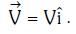The instantaneous oscillating electric field of this em wave is along +y axis. Then the direction of oscillating magnetic field of the em wave will be along :-    (2018)
A: –z direction
B: +z direction
C: –y direction
D: –x direction
Ans:
B
Solution: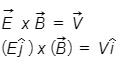(Therefore, electric field vector is along + y axis)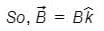i.e., direction of magnetic filed vector is along + z direction.

Q.6. In an electromagnetic wave in free space the root mean square value of the electric field is Erms = 6V/m. The peak value of the magnetic field is :-    (2017)
A: 2.83 × 10–8 T
B: 0.70 × 10–8 T
C: 4.23 × 10–8 T
D: 1.41 × 10–8 T
Ans: A
Solution: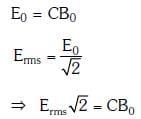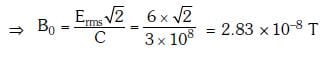Q.7. Out of the following options which one can be used to produce a propagating electromagnetic wave ?    (2016)
A: An accelerating charge
B: A charge moving at constant velocity
C: A stationary charge
D: A charge less particle
Ans:
A
Solution:

An electric charge at rest has electric field in the region around it, but magnetic field is absent. A moving charge produces both electric and magnetic field. When a charge is moving with constant velocity, electric and magnetic field will not change with time. Therefore, no electromagnetic wave will be produced.
But, if the charge is moving with non-zero acceleration, electric and magnetic field will change with space and time. Electromagnetic waves will be produced.
Therefore, accelerated charge emits electromagnetic waves.

The document NEET Previous Year Questions (2014-21): Electromagnetic Waves Notes | Study Physics Class 12 - NEET is a part of the NEET Course Physics Class 12.
All you need of NEET at this link: NEETUse Code STAYHOME200 and get INR 200 additional OFF

## Physics Class 12

134 videos|388 docs|213 tests

### How to Prepare for NEET

Read our guide to prepare for NEET which is created by Toppers & the best Teachers

Track your progress, build streaks, highlight & save important lessons and more!

,

,

,

,

,

,

,

,

,

,

,

,

,

,

,

,

,

,

,

,

,

;